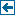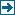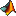Master index Index for Applications/Horace/@d3d# Index for iFit/Applications/Horace/@d3d

## Matlab files in this directory:IXTdataset_3d Convert d3d object into IXTdataset_3dIX_dataset_3d Convert d3d object into IX_dataset_3dacos Implement acos(w1) for objectsacosh Implement acosh(w1) for objectsacot Implement acot(w1) for objectsacoth Implement acoth(w1) for objectsacsc Implement acsc(w1) for objectsacsch Implement acsch(w1) for objectsasec Implement asec(w1) for objectsasech Implement asech(w1) for objectsasin Implement asin(w1) for objectsasinh Implement asinh(w1) for objectsatan Implement atan(w1) for objectsatanh Implement atanh(w1) for objectscalculate_q_bins Calculate qh,qk,ql,en for the centres of the bins of a d3d datasetcalculate_qw_bins Calculate qh,qk,ql,en for the centres of the bins of a d3d datasetchange_crystal Change the crystal lattice and orientation of a d3d object or array of objectscompact Squeezes the data range in a d2d object to eliminate empty binscos Implement cos(w1) for objectscosh Implement cosh(w1) for objectscot Implement acot(w1) for objectscoth Implement acoth(w1) for objectscsc Implement csc(w1) for objectscsch Implement csch(w1) for objectscut Take a cut from a d3d object or array of objects by integrating over one or more of the plot axes.d3d Create a 3D Horace dataset ('d3d')dimensions Find number of dimensions and extent along each dimension ofdisp2sqw_eval Calculate sqw for a model scattering functiondispersion Calculate dispersion relation for dataset or array of datasets.display Display sqw object to screenexp Implement exp(w1) for objectsfit Fits a function to an object, with an optional background function.fit_func Fits a function to an object, with an optional background function.fit_sqw Fit a model for S(Q,w) to an object, with an optional background function.fit_sqw_sqw Fit a model for S(Q,w) to an object, with an optional background function that is also a model for S(Q,w)func_eval Evaluate a function at the plotting bin centres of d3d object or array of d3d objectsget Get a named field from an object, or a structure with allhead Display a summary of a d3d object or file containing d3d information.isvalid Check fields for data_array objectlog Implement log(w1) for objectslog10 Implement log(w1) for objectsmask Remove the bins indicated by the mask arraymask_points Determine the points to keep on the basis of ranges and mask array.minus Implement w1 - w2 for objectsmldivide Implement w1 \ w2 for objectsmpower Implement w1 ^ w2 for objectsmrdivide Implement w1 / w2 for objectsmtimes Implement w1 * w2 for objectsmultifit Simultaneously fit a function to an array of objects.multifit_func Simultaneously fit a function to an array of objects.multifit_sqw Simultaneously fit a model for S(Q,w) to an array of objects.multifit_sqw_sqw Simultaneously fit a model for S(Q,w) to an array of objects.permute Permute the order of the display axes. Syntax the same as the matlab array permute functionplus Implement w1 + w2 for objectsread Read d3d object from a file or array of d3d objects from a set of filesrefine_crystal Refine crystal orientation and lattice parameters for a d3d object.replicate Make a higher dimensional dataset from a three dimensional dataset bysave Save a sqw object to filesave_xye Saves a three dimensional dataset to ASCII filesec Implement sec(w1) for objectssech Implement sech(w1) for objectssection Takes a section out of a 3-dimensional dataset.set Set functionsigvar Create sigvar objectsigvar_get Get signal and variance from object, and a logical array of which values to ignoresigvar_set Set output object signal and variance fields from input sigvar objectsigvar_size Matlab size of signal arraysin Implement sin(w1) for objectssinh Implement sinh(w1) for objectssmooth Smooths a three dimensional datasetsqrt Implement sqrt(w1) for objectssqw Convert input dnd-type object into sqw objectsqw_eval Calculate sqw for a model scattering functionstruct A Comprehensive Guide to Object Oriented Programming in MATLABsubsasgn A Comprehensive Guide to Object Oriented Programming in MATLABsubsref A Comprehensive Guide to Object Oriented Programming in MATLABtan Implement tan(w1) for objectstanh Implement tanh(w1) for objectsuminus Implement -w1 for objectsuplus Implement +w1 for objects

Generated on Mon 26-Nov-2018 15:08:24 by m2html © 2005. iFit (c) E.Farhi/ILL EUPL 1.1